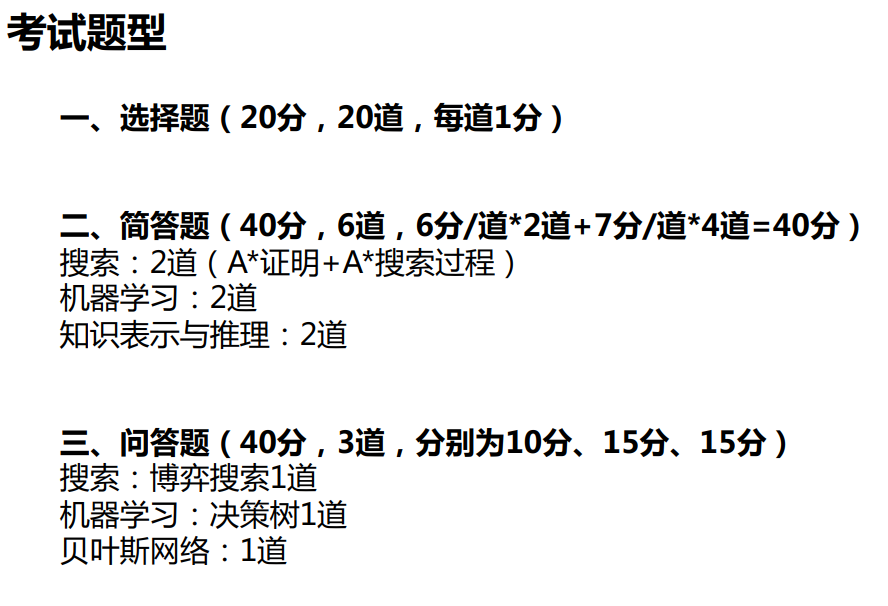# 启发式搜索

$$f(n)=g(n)+h(n)\\ f(n):评价函数\\ h(n):启发函数\\ g^*(n):从起点S到当前点n的最短路径的耗散值\\ h^*(n)从当前点n到目标节点T的最短路径的耗散值\\ f^*(n)=g^*(n)+h^*(n)\\ 带星的是实际值，不带的是估计值\\ g(n)不一定等于g^*(n),只有过程中找最近的扩展才是相等的$$

## 证明

### 引理1.2

A*结束前，Open表中必定存在$f(n)\le f^*(S)$

### 定理2

• 由引理1.1，A*如果不结束，则对Open表中所有的n有$f(n)>f^*(S)$

• 由引理1.2，在A*结束前，必存在节点n，使得$f(n)\le f^*(S)$

### 推论1

Open表上任一具有$f(n)<f^*(S)$的节点n，最终都将被A*选作扩展的结点

### 推论2

A*选作扩展的任一节点n，有$f(n)\le f^*(S)$

# 不确定搜索

## 模拟退火

$$P(Accept)=exp(\frac{-\Delta x}{T})$$

# 约束满足

• 向前查看：约束传播 把能确定的一次性全搞掉，判断邻居是否有合法解
• 启发式：用已知信息决定哪个点先赋值。有多种排序方式，适应不同问题

# 机器学习

## 决策树

$$H(D)=-\sum _ {k=1}^ {|y|}p_k\lg(p_k)$$

$$G(D,a)=H(D)-\sum _ {v=1} ^ V\frac{|D^v|}{|D|}\cdot H(D^v)$$

## 深度学习

### CNN计算

x层卷y层池化配合成一个卷积快，z个卷积块之后交给常规的全连接

Reward Is Enough

A动作空间

X状态空间

R奖赏函数

P状态转移概率

# 知识计算

## 知识表示

$$\text{argmin} \sum_{r\in KB}f_r(h,t)$$

### 基于距离的模型

SE模型 两个实体属于同一个三元组时，向量表示在投影后的空间应该比较靠近

TransE

## 贝叶斯网络

$$P(B_i|A)= \frac {P(B_i) P(A|B_i)}{\sum _ {k=1}^n P(B_k)P(A|B_k)}$$